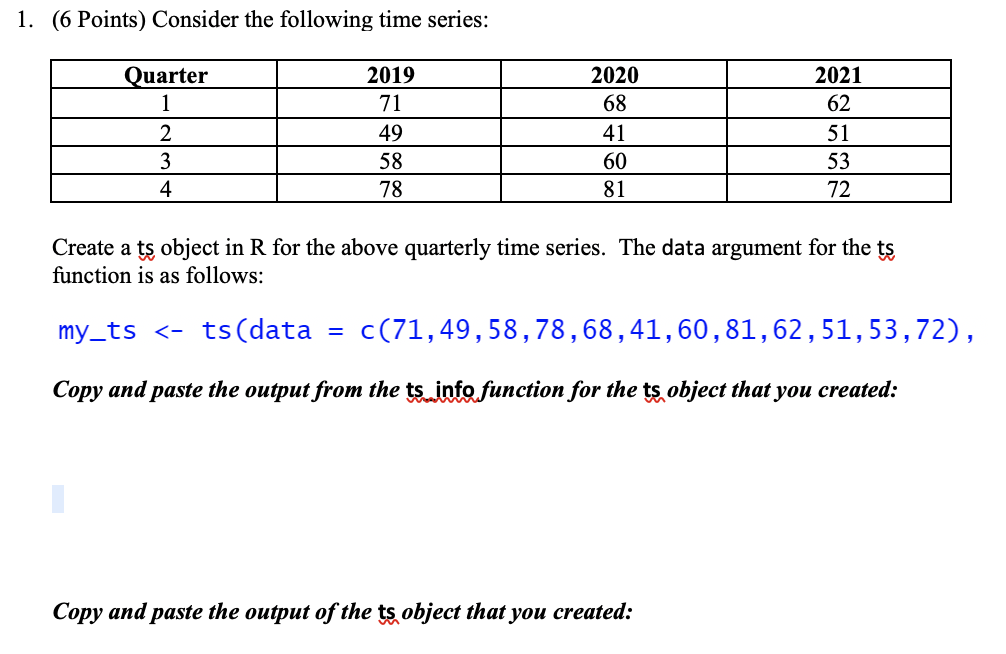Home / Expert Answers / Statistics and Probability / 1-6-points-consider-the-following-time-series-create-a-ts-object-in-mathrm-r-for-the-a-pa233

# (Solved): 1. (6 Points) Consider the following time series: Create a ts object in $$\mathrm{R}$$ for the a ...1. (6 Points) Consider the following time series: Create a ts object in $$\mathrm{R}$$ for the above quarterly time series. The data argument for the ts function is as follows: my_ts <- ts $$($$ data $$=c(71,49,58,78,68,41,60,81,62,51,53$$, Copy and paste the output from the ts info function for the ts object that you created: Copy and paste the output of the ts object that you created:

We have an Answer from Expert